# Graphs: Types, Examples & Functions

An error occurred trying to load this video.

Try refreshing the page, or contact customer support.

Coming up next: Congruent in Math: Definition & Examples

### You're on a roll. Keep up the good work!

Replay
Your next lesson will play in 10 seconds
• 0:01 Different Types of Graphs
• 0:32 Linear and Power Graphs
• 2:00 Quadratic and…
• 3:12 Rational and…
• 3:53 Logarithmic and…
• 4:21 Lesson Summary

Want to watch this again later?

Timeline
Autoplay
Autoplay
Speed

#### Recommended Lessons and Courses for You

Lesson Transcript
Instructor: Yuanxin (Amy) Yang Alcocer

Amy has a master's degree in secondary education and has taught math at a public charter high school.

In this lesson, learn how you can differentiate from the eight most common types of functions and their graphs. Learn the distinct look of each so you can easily distinguish them from each other.

## Different Types of Graphs

There are eight types of graphs that you will see more often than other types. Each has its own type of function that produces the graphs. They are easy to visually distinguish and by knowing how each looks, you can get an idea of what a graph might look like just by analyzing the function. The eight types are linear, power, quadratic, polynomial, rational, exponential, logarithmic, and sinusoidal.

## Linear Graphs

Linear graphs are produced by linear functions of this form: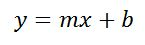Linear functions have variables to the first degree and have two constants that determine the location of the graph. These functions always graph into a line. The constant m determines whether the line slopes down or up. If it is positive, the line will slope up, and if it is negative, then the line will slope down.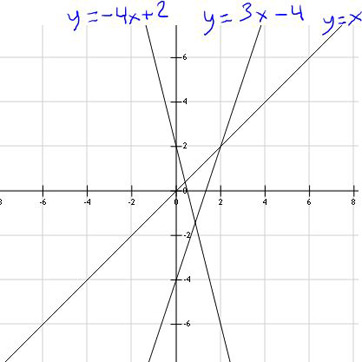## Power Graphs

Power graphs are produced by functions with only one term and a power. The power can be positive, negative, or even a fraction.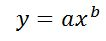The graphs that these types of functions produce vary depending on the power. If the power is positive, the graph changes direction based on the number of the power. If the power is even, the graph will have both edges going in the same direction. If the power is odd, the graph will have one edge going up and another going down. If the power is negative, it will have two parts. Each part will avoid the x=0 line because that will cause division by zero. When the power is a fraction, the graph goes up at x=0 and then when y is positive, it starts curving towards the x-axis.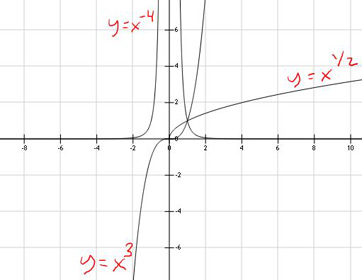Quadratics are functions where the highest power is two.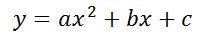They graph into parabolas. The constants a, b, and c determine the location of the parabola on the graph. The a tells you whether the parabola will open up or down. If it's positive, it will open up and smile. If it's negative, it will open down and frown.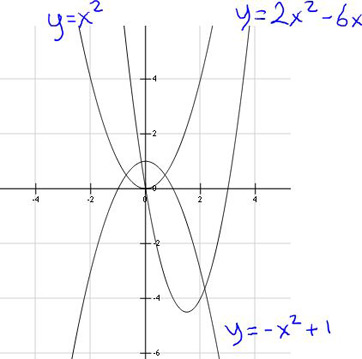## Polynomial Graphs

Polynomials are a more general function than a quadratic and allow for higher powers that are still whole numbers.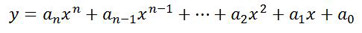These functions produce more interesting graphs with more curves. The highest power of the function tells you how many curves or ups and downs the graph may have.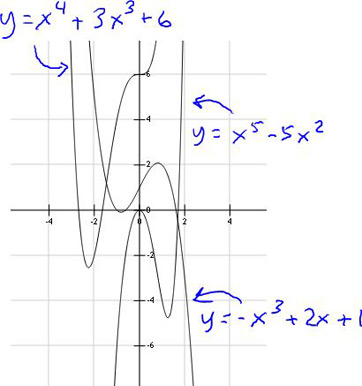To unlock this lesson you must be a Study.com Member.

### Register to view this lesson

Are you a student or a teacher?

### Unlock Your Education

#### See for yourself why 30 million people use Study.com

##### Become a Study.com member and start learning now.
Back
What teachers are saying about Study.com

### Earning College Credit

Did you know… We have over 160 college courses that prepare you to earn credit by exam that is accepted by over 1,500 colleges and universities. You can test out of the first two years of college and save thousands off your degree. Anyone can earn credit-by-exam regardless of age or education level.